Notebook to reproduce the analysis reported in "Outliers Exclusion Procedures Must be Blind to the Researcher’s Hypothesis."

# Preamble¶

## Imports¶

Show Code
import multiprocessing
from functools import partial

import matplotlib.patches as mpatches
import matplotlib.pyplot as plt
import numpy as np
import pandas as pd
import pingouin as pg
import scipy.stats as stats
import seaborn as sns
import statsmodels.api as sm
import statsmodels.formula.api as smf
from IPython.display import HTML, display
from joblib import Parallel, delayed
from statsmodels.stats.outliers_influence import OLSInfluence
from tqdm import tqdm


## Functions¶

Show Code
# Misc Functions
def welsch_pval(x, y):
"""
Return the p-value of a Welsch t-test of the difference between two vectors x and y.
"""
return stats.ttest_ind(x, y, equal_var=False).pvalue

def mw_pval(x, y):
"""
Return the p-value of a Mann-Whitney test of the difference between two vectors x and y.
"""
return stats.mannwhitneyu(x, y, alternative="two-sided").pvalue

def ks_pval(x, y):
"""
Return the p-value of a Kolmogorov-Smirnov test of the difference between two vectors x and y.
"""
return stats.ks_2samp(x, y, alternative="two-sided").pvalue

def mixturesampler(size):
"""
Return a mixture of two normal distribution: The value is sampled from N(0, 1) with p = .95,
and with N(5, 1) with p= .05, such that 5% of observations are outliers.
"""
low = np.random.normal(0, 1, size)
high = np.random.normal(5, 1, size)
mixture = np.random.choice([0, 1], p=[0.95, 0.05], size=size)
return low + high * mixture

def categoricalsampler(size):
"""
Return a dummy variable vector of size N
"""
return np.repeat([-1, 1], int(size / 2))

# Functions to remove outliers in discrete cell designs
def remove_outliers(x, cutoff_type="IQR", cutoff_thresh=2):
"""
Exclude outliers from a vector.
"""
x = x.copy()
if cutoff_type == "IQR":
iqr = stats.iqr(x)
low = np.percentile(x, 25) - cutoff_thresh * iqr
high = np.percentile(x, 75) + cutoff_thresh * iqr
return x[(x >= low) & (x <= high)]
elif cutoff_type == "z-score":
sd = np.std(x)
low = np.mean(x) - cutoff_thresh * sd
high = np.mean(x) + cutoff_thresh * sd
return x[(x >= low) & (x <= high)]
elif cutoff_type == "MAD":
mad = stats.median_abs_deviation(x, scale="normal")
med = np.median(x)
low = med - cutoff_thresh * mad
high = med + cutoff_thresh * mad
return x[(x >= low) & (x <= high)]
elif cutoff_type == "WINS":
sd = np.std(x)
low = np.mean(x) - cutoff_thresh * sd
high = np.mean(x) + cutoff_thresh * sd
x[x <= low] = low
x[x >= high] = high
return x
else:
raise ValueError(
f"Cutoff type {cutoff_type} not understood. Must be 'IQR' or 'SD'"
)

def remove_outliers_common(x, y, cutoff_type="IQR", cutoff_thresh=2):
"""
Exclude outliers from two vectors, using the same cutoff for both.
"""
xy = np.concatenate([x, y])
x = x.copy()
y = y.copy()
if cutoff_type == "IQR":
iqr = stats.iqr(xy)
low = np.percentile(xy, 25) - cutoff_thresh * iqr
high = np.percentile(xy, 75) + cutoff_thresh * iqr
return (
x[(x >= low) & (x <= high)],
y[(y >= low) & (y <= high)],
)

elif cutoff_type == "z-score":
sd = np.std(xy)
low = np.mean(xy) - cutoff_thresh * sd
high = np.mean(xy) + cutoff_thresh * sd
return (
x[(x >= low) & (x <= high)],
y[(y >= low) & (y <= high)],
)

elif cutoff_type == "MAD":
mad = stats.median_abs_deviation(x, scale="normal")
med = np.median(x)
low = med - cutoff_thresh * mad
high = med + cutoff_thresh * mad
return (
x[(x >= low) & (x <= high)],
y[(y >= low) & (y <= high)],
)

elif cutoff_type == "WINS":
sd = np.std(xy)
low = np.mean(xy) - cutoff_thresh * sd
high = np.mean(xy) + cutoff_thresh * sd
x[x <= low] = low
x[x >= high] = high
y[y <= low] = low
y[y >= high] = high
return x, y
else:
raise ValueError(
f"Cutoff type {cutoff_type} not understood. Must be 'IQR' or 'SD'"
)

def remove_outliers_iter(x, cutoff_type="IQR", cutoff_thresh=2):
"""
Iteratively exclude outliers from two vectors until none are found.
"""
x = x.copy()
if cutoff_type != "WINS":
x_before = x
while True:
x_after = remove_outliers(x_before, cutoff_type, cutoff_thresh)
if x_before.shape == x_after.shape:
return x_after
else:
x_before = x_after
else:
x_before = x
while True:
x_after = remove_outliers(x_before, cutoff_type, cutoff_thresh)
if (x_before == x_after).mean() == 1:
return x_after
else:
x_before = x_after

# Functions to remove residual-based outliers
def remove_residuals_blind(y, X, cutoff_thresh=2):
"""
Remove outliers from residuals of model that is blind to the key predictor X.
"""
model = sm.OLS(y, X[:, 1:])
results = model.fit()
resids = OLSInfluence(results).resid_studentized_internal
return (
y[(resids >= -cutoff_thresh) & (resids <= cutoff_thresh)],
X[(resids >= -cutoff_thresh) & (resids <= cutoff_thresh), :],
)

def remove_residuals_aware(y, X, cutoff_thresh=2):
"""
Remove outliers from residuals of model that is aware of the key predictor X.
"""
model = sm.OLS(y, X)
results = model.fit()
resids = OLSInfluence(results).resid_studentized_internal
return (
y[(resids >= -cutoff_thresh) & (resids <= cutoff_thresh)],
X[(resids >= -cutoff_thresh) & (resids <= cutoff_thresh), :],
)

# Functions comparing results under different exclusion strategies
def compare_pvals_under_exclusions(x, y, cutoff_type="IQR", cutoff_thresh=2, test=None):
"""
Compare two vectors of data and return the p-values when (1) No outliers are excluded, (2) Outliers
are excluded based on a common cutoff, (3) Outliers are excluded using
a condition-specific cutoff, and (4) Outliers are recursively excluded.
"""
p = test(x, y)

x_common, y_common = remove_outliers_common(x, y, cutoff_type, cutoff_thresh)
p_common = test(x_common, y_common)

x_diff = remove_outliers(x, cutoff_type, cutoff_thresh)
y_diff = remove_outliers(y, cutoff_type, cutoff_thresh)
p_diff = test(x_diff, y_diff)

x_diff_iter = remove_outliers_iter(x, cutoff_type, cutoff_thresh)
y_diff_iter = remove_outliers_iter(y, cutoff_type, cutoff_thresh)
p_diff_iter = test(x_diff_iter, y_diff_iter)
return [p, p_common, p_diff, p_diff_iter]

def compare_tstats_under_exclusions(x, y, cutoff_type="IQR", cutoff_thresh=2):
"""
Compare two vectors of data and return the t-stats when (1) Outliers
are excluded based on a common cutoff or (2) Outliers are excluded using
a condition-specific cutoff
"""
x_common, y_common = remove_outliers_common(x, y, cutoff_type, cutoff_thresh)
diff_common = stats.ttest_ind(x_common, y_common).statistic

x_diff = remove_outliers(x, cutoff_type, cutoff_thresh)
y_diff = remove_outliers(y, cutoff_type, cutoff_thresh)
diff_diff = stats.ttest_ind(x_diff, y_diff).statistic
return [diff_common, diff_diff]

def compare_pvals_under_resid_exclusions(y, X, cutoff_thresh=2):
"""
Compare p-values from OLS model when (1) No outliers are excluded, (2) Outliers
are excluded based the hypothesis-blind residuals,
(3) Outliers excluded based the hypothesis-aware residuals
"""
y_blind, X_blind = remove_residuals_blind(y, X, cutoff_thresh=cutoff_thresh)
y_aware, X_aware = remove_residuals_aware(y, X, cutoff_thresh=cutoff_thresh)
pvals_base = sm.OLS(y, X).fit().pvalues
pvals_blind = sm.OLS(y_blind, X_blind).fit().pvalues
pvals_aware = sm.OLS(y_aware, X_aware).fit().pvalues
return pvals_base, pvals_blind, pvals_aware

# Functions simulating exclusion impacts in discrete designs.
def simulate_exclusion_impact(n_boots, seed=2743892347):
"""
Simulate the impact of exclusions based on descriptive statistics
in all the different experimental setups.
"""

# Seed for reproducibility
np.random.seed(seed)

# Three data samplers
data_samplers = [np.random.normal, np.random.lognormal, mixturesampler]
dic_samplers = {0: "Normal", 1: "Log-Normal", 2: "Normal Mixture"}

# Three different sample sizes
sample_sizes = [50, 100, 250]
dic_ssizes = {0: 50, 1: 100, 2: 250}

# Three different tests
tests = [welsch_pval, mw_pval, ks_pval]
dic_testtypes = {0: "Welsch's t", 1: "Mann-Whitney", 2: "K-S"}

# Three cutoff types:
cuttypes = ["IQR", "z-score", "MAD"]
cuttypes_labels = ["IQR Distance", "z-score", "MAD"]
dic_cuttypes = {i: c for i, c in enumerate(cuttypes_labels)}

# Three different exclusion thresholds
cutthreshs = [1.5, 2, 3]
dic_cutthreshs = {i: c for i, c in enumerate(cutthreshs)}

# Four outliers exclusion strategy
dic_exclutypes = {
0: "None",
1: "Across",
2: "Within",
3: "Within, Iterated",
}

def gen_data_and_get_pvals(seed):
"""
A function that will be parallelized for faster results.
"""
np.random.seed(seed)
data_samplers = [np.random.normal, np.random.lognormal, mixturesampler]
pvs = np.empty(shape=(3, 3, 3, 3, 3, 4))  # Empty array to store the p-values
for j, samp in enumerate(data_samplers):  # For each data type
for k, n in enumerate(sample_sizes):  # For each sample size
x, y = samp(size=(2, n))  # Draw two vectors from the data
for l, test in enumerate(tests):  # For each of the tests
for m, cuttype in enumerate(
cuttypes
):  # For each of the cutoff types
for n, cutthresh in enumerate(
cutthreshs
):  # For each of the cutoff thresholds
p = compare_pvals_under_exclusions(
x,
y,
test=test,
cutoff_type=cuttype,
cutoff_thresh=cutthresh,
)
pvs[j, k, l, m, n, :] = p
return pvs

seeds = np.random.randint(
0, 2147483647, size=n_boots
)  # Seeds sent to parallelized functions
num_cores = multiprocessing.cpu_count()  # Number of cores
# Parallelize bootstrap across cores
results = Parallel(n_jobs=num_cores)(
delayed(gen_data_and_get_pvals)(s) for s in tqdm(seeds)
)
pvals = np.array(results)
# Reshape the results into a square matrix
cols = np.column_stack(
list(map(np.ravel, np.meshgrid(*map(np.arange, pvals.shape), indexing="ij")))
+ [pvals.ravel()]
)
# Store into a dataframe
df_pvals = pd.DataFrame(
cols,
columns=[
"nboot",
"Data",
"N",
"Test",
"Method",
"Threshold",
"Level",
"pvalue",
],
)
# Add descriptive labels to each of the setups
df_pvals["Data"] = df_pvals.Data.map(dic_samplers)
df_pvals["N"] = df_pvals.N.map(dic_ssizes)
df_pvals["Test"] = df_pvals.Test.map(dic_testtypes)
df_pvals["Method"] = df_pvals.Method.map(dic_cuttypes)
df_pvals["Threshold"] = df_pvals.Threshold.map(dic_cutthreshs)
df_pvals["Level"] = pd.Categorical(
df_pvals.Level.map(dic_exclutypes),
categories=["None", "Across", "Within", "Within, Iterated"],
)
# Compute Type I error rates for different alphas
df_pvals["$\\alpha_{{05}}$"] = (df_pvals.pvalue < 0.05) * 1
df_pvals["$\\alpha_{{01}}$"] = (df_pvals.pvalue < 0.01) * 1
df_pvals["$\\alpha_{{001}}$"] = (df_pvals.pvalue < 0.001) * 1
return df_pvals

def simulate_resid_exclusion_impact(n_boots, seed=2743892347):
"""
Simulate the impact of exclusions based on residuals
in all the different experimental setups.
"""

# Seed for reproducibility
np.random.seed(seed)

# Three data samplers for IV
DV_samplers = [np.random.normal, np.random.lognormal, mixturesampler]
dic_DV_samplers = {0: "Normal", 1: "Log-Normal", 2: "Normal Mixture"}

# Two data samplers for IV
IV_samplers = [np.random.normal, categoricalsampler]
dic_IV_samplers = {0: "Continuous", 1: "Categorical"}

# Three different sample sizes
sample_sizes = [50, 100, 240]
dic_ssizes = {0: 50, 1: 100, 2: 240}

# Four outliers exclusion strategy
dic_exclutypes = {0: "None", 1: "Predictor-Blind", 2: "Predictor-Aware"}

def gen_data_and_get_pvals(seed):
"""
A function that will be parallelized for faster results.
"""
np.random.seed(seed)
DV_samplers = [np.random.normal, np.random.lognormal, mixturesampler]
IV_samplers = [np.random.normal, categoricalsampler]
# Empty array to store the p-values
pvals = np.empty(shape=(3, 2, 3, 3))
for j, dvsamp in enumerate(DV_samplers):  # For each DV type
for k, ivsamp in enumerate(IV_samplers):  # For each IV type
for l, n in enumerate(sample_sizes):  # For each sample size
intercept = np.ones(n)
w = np.random.normal(size=n)  # Vector of covariates
y = dvsamp(size=n) + w  # Vector of DV, influenced by covariates
x = ivsamp(size=n)  # Vector of IV, that has no influence on Y.
X = np.vstack([x, intercept, w]).T
pvals[j, k, l, :] = compare_pvals_under_resid_exclusions(y, X)
return pvals

seeds = np.random.randint(
0, 2147483647, size=n_boots
)  # Seeds sent to parallelized functions
num_cores = multiprocessing.cpu_count()  # Number of cores
# Parallelize bootstrap across cores
results = Parallel(n_jobs=num_cores)(
delayed(gen_data_and_get_pvals)(s) for s in tqdm(seeds)
)
pvals = np.array(results)

# Reshape the results into a square matrix
cols = np.column_stack(
list(map(np.ravel, np.meshgrid(*map(np.arange, pvals.shape), indexing="ij")))
+ [pvals.ravel()]
)
# Store into a dataframe
df_pvals = pd.DataFrame(
cols, columns=["nboot", "DVType", "IVType", "N", "Level", "pvalue",],
)
# Add descriptive labels to each of the setups
df_pvals["DVType"] = df_pvals.DVType.map(dic_DV_samplers)
df_pvals["IVType"] = df_pvals.IVType.map(dic_IV_samplers)
df_pvals["N"] = df_pvals.N.map(dic_ssizes)
df_pvals["Level"] = pd.Categorical(
df_pvals.Level.map(dic_exclutypes),
categories=["None", "Predictor-Blind", "Predictor-Aware"],
)
# Compute Type I error rates for different alphas
df_pvals["$\\alpha_{{05}}$"] = (df_pvals.pvalue < 0.05) * 1
df_pvals["$\\alpha_{{01}}$"] = (df_pvals.pvalue < 0.01) * 1
df_pvals["$\\alpha_{{001}}$"] = (df_pvals.pvalue < 0.001) * 1
return df_pvals

# Functions to simulate exclusion impacts in real data
def simulate_exclusion_impact_ckg(df, n_boots, n=31, seed=2743892347):
"""
Simulate the exclusion impacts in Cao, Kong, and Galinsky's original data.
This function shuffles the residuals of the model on the full data (before exclusions)
to create a synthetic dataset, and compares two random groups of size N draw from this data.
N = 31 for Study 2, and 37 for Study 1.
"""
# Predicted values before excluding participants.
predicted = smf.ols("ParetoEfficiency~C(Condition)", data=df).fit().predict()

# Residuals
resids = (df.ParetoEfficiency - predicted).values

# Seed for reproducibility
np.random.seed(seed)  # Seed for reproducibility

# Three cutoff types:
cuttypes = ["IQR", "z-score", "MAD"]
cuttypes_labels = ["IQR Distance", "z-score", "MAD"]
dic_cuttypes = {i: c for i, c in enumerate(cuttypes_labels)}

# Three different exclusion thresholds
cutthreshs = [1.5, 2, 3]
dic_cutthreshs = {i: c for i, c in enumerate(cutthreshs)}

# Four different exclusion types
dic_exclutypes = {
0: "None",
1: "Across",
2: "Within",
3: "Within, Iterated",
}

def gen_data_and_get_pvals(seed):
"""
A function that will be parallelized for faster results.
"""
np.random.seed(seed)
# Empty array to store the p-values
pvals = np.empty(shape=(3, 3, 4))
np.random.shuffle(resids)  # Shuffle the residuals
data = (
predicted + resids
)  # Add residuals to predicted values to generate synthetic data
xy = np.random.choice(
data, n * 2, replace=False
)  # Draw a random sample from the data
x, y = xy[:n], xy[n:]  # Split them in two "conditions"
for j, cuttype in enumerate(cuttypes):  # For each cutoff type
for k, cutthresh in enumerate(cutthreshs):  # For each threshold
pvals[
j, k, :
] = compare_pvals_under_exclusions(  # Compare p-values under different exclusions
x,
y,
cutoff_thresh=cutthresh,
cutoff_type=cuttype,
test=welsch_pval,
)
return pvals

seeds = np.random.randint(
0, 2147483647, size=n_boots
)  # Seeds sent to parallelized functions

num_cores = multiprocessing.cpu_count()  # Number of cores
# Parallelize bootstrap across cores
results = Parallel(n_jobs=num_cores)(
delayed(gen_data_and_get_pvals)(s) for s in tqdm(seeds)
)
pvals = np.array(results)

# Reshape the results into a square matrix
cols = np.column_stack(
list(map(np.ravel, np.meshgrid(*map(np.arange, pvals.shape), indexing="ij")))
+ [pvals.ravel()]
)

# Store the matrix as a dataframe
df_pvals = pd.DataFrame(
cols, columns=["nboot", "Method", "Threshold", "Level", "pvalue"],
)

# Add descriptive labels
df_pvals["Method"] = df_pvals.Method.map(dic_cuttypes)
df_pvals["Threshold"] = df_pvals.Threshold.map(dic_cutthreshs)
df_pvals["Level"] = pd.Categorical(
df_pvals.Level.map(dic_exclutypes),
categories=["None", "Across", "Within", "Within, Iterated"],
)

# Compute Type I error rates for different alphas
df_pvals["$\\alpha_{{05}}$"] = (df_pvals.pvalue < 0.05) * 1
df_pvals["$\\alpha_{{01}}$"] = (df_pvals.pvalue < 0.01) * 1
df_pvals["$\\alpha_{{001}}$"] = (df_pvals.pvalue < 0.001) * 1
return df_pvals

# Plotting functions
def hist_no_edge(x, *args, **kwargs):
"""
Plot a histogram without the left and right edges. Useful for survival curves.
"""
bins = kwargs.pop("bins")
cumulative = kwargs.pop("cumulative", False)
# Add a bin for all p-values between the upper bin and 1.
cnt, edges = np.histogram(x, bins=bins.tolist() + )
ax = plt.gca()
if cumulative:
prop = cnt.cumsum() / cnt.sum()
ax.step(edges[:-2], prop[:-1], *args, **kwargs)
else:
ax.step(edges[:-2], cnt, *args, **kwargs)

def plot_simulation_results(data, include_iterated=True):
"""
Plot the survival curve of all the simulations.
"""
gb = data.groupby(["Threshold", "Method", "Level"])  # Data by group.

# Type I error at various alpha levels
p_05 = gb["$\\alpha_{{05}}$"].mean()
p_01 = gb["$\\alpha_{{01}}$"].mean()
p_001 = gb["$\\alpha_{{001}}$"].mean()

# Labels for lines and panels
thresholds = [1.5, 2.0, 3.0]
methods = ["IQR Distance", "z-score", "MAD"]
if include_iterated:
levels = ["None", "Across", "Within", "Within, Iterated"]
labels = ["None", "Across", "Within", "Within, Iter."]
maxcoord = 4
else:
levels = ["None", "Across", "Within"]
labels = ["None", "Across", "Within"]
data = data.query("Level != 'Within, Iterated'")
maxcoord = 3

ycoords = 0.65 + np.arange(0, 1, 0.06)  # Coordinates to plot legend

pal = sns.color_palette()[0:4]  # Colors

# Initialize the plot
g = sns.FacetGrid(
data,
col="Threshold",
row="Method",
hue="Level",
hue_order=levels,
col_order=thresholds,
row_order=methods,
height=3.4,
aspect=1.2,
sharey=True,
sharex=True,
hue_kws=dict(ls=[(0, ()), (1, (5, 5)), (0, ()), (1, ())]),
)

# Map the survival curve to each panel and color
g.map(
hist_no_edge,
"pvalue",
cumulative=True,
bins=np.logspace(np.log10(10e-40), np.log10(0.05), 10000),
lw=1.5,
)

# Change the axes labels
g.set_ylabels("Fraction of Sig. Tests ($p < \\alpha$)")
g.set_xlabels("Sig. Threshold ($\\alpha$)")
g.set_titles("Cutoff: {col_name} * {row_name}")

# Adjust the axes and ticks
for i, (axe, meth) in enumerate(zip(g.axes, methods)):
for j, (ax, thresh) in enumerate(zip(axe, thresholds)):
ax.set_xlim(10e-8, 0.05)
ax.set_xscale("log")
ax.set_xticks([10e-8, 10e-7, 10e-6, 10e-5, 10e-4, 10e-3, 0.05])
ax.set_xticklabels(
["10e-8", "10e-7", ".00001", ".0001", ".001", ".01", ".05"]
)
ax.set_xticks([], minor=True)
ax.invert_xaxis()
ax.annotate(
f"Type I Error Rate at $[\\alpha_{{05}}, \\alpha_{{01}}, \\alpha_{{001}}]$",
(1, ycoords[maxcoord]),
color="black",
fontsize=10,
ha="right",
xycoords="axes fraction",
)
_, yh = ax.get_ylim()
ys = np.round(yh / 6, 2)
ax.set_yticks(np.arange(0, yh, ys))
# Add the false-positive annotations
for k, (lab, lev) in enumerate(zip(labels, levels)):
ax.annotate(
f"{lab}: [{p_05.loc[thresh, meth, lev]:.2f}, "
f" {p_01.loc[thresh, meth, lev]:.2f}, "
f"{p_001.loc[thresh, meth, lev]:.3f}]",
(1, ycoords[k]),
color=pal[k],
ha="right",
xycoords="axes fraction",
fontsize=10,
)

def plot_resids_simulation_results(data, include_iterated=True):
"""
Plot the survival curve of all the simulations.
"""
gb = data.groupby(["DVType", "IVType", "Level"])  # Data by group.

# Type I error at various alpha levels
p_05 = gb["$\\alpha_{{05}}$"].mean()
p_01 = gb["$\\alpha_{{01}}$"].mean()
p_001 = gb["$\\alpha_{{001}}$"].mean()

# Labels for lines and panels
dvtype_labels = ["Normal", "Log-Normal", "Normal Mixture"]
ivtype_labels = ["Continuous", "Categorical"]

levels = ["None", "Predictor-Blind", "Predictor-Aware"]
labels = ["None", "Predictor-Blind", "Predictor-Aware"]
maxcoord = 3

ycoords = 0.65 + np.arange(0, 1, 0.06)  # Coordinates to plot legend

pal = sns.color_palette()[0:4]  # Colors

# Initialize the plot
g = sns.FacetGrid(
data,
col="DVType",
row="IVType",
hue="Level",
hue_order=levels,
col_order=dvtype_labels,
row_order=ivtype_labels,
height=3.4,
aspect=1.2,
sharey=True,
sharex=True,
hue_kws=dict(ls=[(0, ()), (1, (5, 5)), (0, ()), (1, ())]),
)

# Map the survival curve to each panel and color
g.map(
hist_no_edge,
"pvalue",
cumulative=True,
bins=np.logspace(np.log10(10e-40), np.log10(0.05), 10000),
lw=1.5,
)

# Change the axes labels
g.set_ylabels("Fraction of Sig. Tests ($p < \\alpha$)")
g.set_xlabels("Sig. Threshold ($\\alpha$)")
g.set_titles("{col_name} Data, {row_name} IV")

# Adjust the axes and ticks
for i, (axe, ivtyp) in enumerate(zip(g.axes, ivtype_labels)):
for j, (ax, dvtyp) in enumerate(zip(axe, dvtype_labels)):
ax.set_xlim(10e-8, 0.05)
ax.set_xscale("log")
ax.set_xticks([10e-8, 10e-7, 10e-6, 10e-5, 10e-4, 10e-3, 0.05])
ax.set_xticklabels(
["10e-8", "10e-7", ".00001", ".0001", ".001", ".01", ".05"]
)
ax.set_xticks([], minor=True)
ax.invert_xaxis()
ax.annotate(
f"Type I Error Rate at $[\\alpha_{{05}}, \\alpha_{{01}}, \\alpha_{{001}}]$",
(1, ycoords[maxcoord]),
color="black",
fontsize=10,
ha="right",
xycoords="axes fraction",
)
_, yh = ax.get_ylim()
ys = np.round(yh / 6, 2)
ax.set_yticks(np.arange(0, yh, ys))
# Add the false-positive annotations
for k, (lab, lev) in enumerate(zip(labels, levels)):
ax.annotate(
f"{lab}: [{p_05.loc[dvtyp, ivtyp, lev]:.2f}, "
f" {p_01.loc[dvtyp, ivtyp, lev]:.2f}, "
f"{p_001.loc[dvtyp, ivtyp, lev]:.3f}]",
(1, ycoords[k]),
color=pal[k],
ha="right",
xycoords="axes fraction",
fontsize=10,
)


## Loading Data from Cao, Kong and Galinsky (2020)¶

Show Code
def flag_outliers_iqr(x, cutoff_thresh=3):
"""
Flag the outliers using the IQR method.
"""
iqr = stats.iqr(x)
low = np.percentile(x, 25) - cutoff_thresh * iqr
high = np.percentile(x, 75) + cutoff_thresh * iqr
return np.array((x <= low) | (x >= high))

def generate_outliers_subsets(df):
"""
Process the data from Cao, Kong, and Galinsky according to different outliers exclusions:
* Before Exclusions
* Exclusions Across Data
* Exclusions Within Conditions
* Exclusions Within Conditions, Iterated
"""

df["Exclusions"] = "None"

# Flagging outliers within and across conditions
df["Outliers_within"] = df.groupby("Condition")["ParetoEfficiency"].transform(
flag_outliers_iqr
)
df["Outliers_across"] = flag_outliers_iqr(df["ParetoEfficiency"])

# Generating different subsets of data:
## ...Outliers removed across
df_oa = df[df.Outliers_across == False].copy()
df_oa["Exclusions"] = "Across"

## ...Outliers removed within
df_ow = df[df.Outliers_within == False].copy()
df_ow["Exclusions"] = "Within"
df_ow["Outliers_within_iter"] = df_ow.groupby("Condition")[
"ParetoEfficiency"
].transform(flag_outliers_iqr)

## ...Outliers removed iteratively
df_owi = df_ow[df_ow.Outliers_within_iter == False].copy()
df_owi["Exclusions"] = "Within, Iterated"

# Return the data with the four possible subsets
return pd.concat([df, df_oa, df_ow, df_owi])

# Study 1 from Cao, Kong and Galinsky
df1 = pd.read_csv("Data/CKG_Study 1.csv")

# Shorter variable names
df1["Outliers"] = (
df1[
"Pareto Efficiency Outlier (1=Yes, 0=No) (for Dyad at least one having prior negotiation training)"
]
== "1"
) * 1
df1["ParetoEfficiency"] = df1["Pareto Efficiency"]

# Processing non-outliers exclusions
df1_clean = df1[
df1[
"Excluded (dyad at least one having prior negotiation training or violating the protocol) (1 = Yes, 0 = No)"
]
== 0
]

# One data point per dyad
df1_clean = df1_clean.drop_duplicates(subset=["Team ID"])[
["Condition", "Outliers", "ParetoEfficiency"]
]

# Generating subsets
df1_subsets = generate_outliers_subsets(df1_clean)
df1_subsets["Study"] = "Study 1"

# Same thing for Study 2
df2 = pd.read_csv("Data/CKG_Study 2.csv")

# Shorter variable names
df2["Outliers"] = (df2["Extreme Outlier (Pareto Efficiency) (1=Yes, 0=No)"] == "1") * 1
df2["ParetoEfficiency"] = df2["Pareto Efficiency"]

# Processing non-outliers exclusions
df2_clean = df2[df2["Exclusion (Both Prior Training and Protocol Violation)"] == 0]

# One data point per dyad
df2_clean = df2_clean.drop_duplicates(subset=["Team ID"])[
["Condition", "Outliers", "ParetoEfficiency"]
]

# Generating subsets
df2_subsets = generate_outliers_subsets(df2_clean)
df2_subsets["Study"] = "Study 2"

df_all = pd.concat([df1_subsets, df2_subsets])


# Figures and Results¶

## Figure 1: Example of a Boxplot¶

Show Code
np.random.seed(4278247)  # Seed for reproducibility

# Random data and conditions
x = 500 - np.random.exponential(size=30) * 100
c = np.repeat(["Treatment", "Control"], 15)
df = pd.DataFrame({"Response": x, "Condition": c})

# Boxplot
with sns.plotting_context("paper", font_scale=1.1):
pal = sns.color_palette()
fig, ax = plt.subplots(1, figsize=(5, 3))
sns.boxplot(x="Condition", y="Response", data=df, order=["Control", "Treatment"])

# Making boxplot transparent
for i, box in enumerate(ax.artists):
box.set_edgecolor(pal[i])
box.set_facecolor("white")
for j in range(6 * i, 6 * (i + 1)):
ax.lines[j].set_color(pal[i])

# Add overlay with observations
sns.stripplot(
x="Condition",
y="Response",
data=df,
ax=ax,
alpha=0.5,
order=["Control", "Treatment"],
)
sns.despine()
ax.set_xlabel("")
plt.savefig("Figures/Fig1.png", dpi=200, bbox_inches="tight")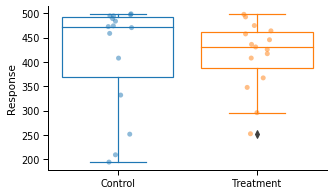## Figure 2: Visualizing the impact of exclusions within conditions¶

Show Code
np.random.seed(273984312)  # Seed for reproducibility

# Generate vectors of Student t-statistics for two outliers exclusion strategies: Across vs. Within Conditions.
data = [
compare_tstats_under_exclusions(
np.random.lognormal(size=100),
np.random.lognormal(size=100),
cutoff_type="IQR",
cutoff_thresh=1.5,
)
for i in range(5000)
]

# Store vectors into a dataframe
df = pd.DataFrame(data, columns=["Across the Data", "Within Conditions"])
df["bootid"] = np.arange(5000)
df_long = pd.melt(
df, id_vars=["bootid"], value_vars=["Across the Data", "Within Conditions"],
)
df_long["Reject H0"] = (np.abs(df_long.value) > stats.t.ppf(0.975, 198)).map(
{False: "No", True: "Yes"}
)

# Plot the histogram of the t-statistics
bins = np.linspace(-5.9161, 5.9161, 70)
maxprop = np.diff(stats.t.cdf(bins, 198)).max()
maxp = stats.t.pdf(0, 198)
scaling = 5000 * (maxprop / maxp)
with sns.plotting_context("paper", font_scale=1.1):
g = sns.FacetGrid(
row="variable",
data=df_long,
aspect=2.5,
height=2.5,
hue="Reject H0",
sharey=True,
)
g.map(sns.histplot, "value", bins=bins, alpha=0.5, stat="count", common_norm=True)

# Add the theoretical Student t-distribution
for ax in g.axes.flatten():
ticks = ax.get_yticks()
ticklabs = np.round(np.linspace(0, 0.4, 5), 2)
ax.set_yticks(ticklabs * scaling)
ax.set_yticklabels(ticklabs)
leg = ax.legend(frameon=False, title="H0 is Rejected?")
ax.add_artist(leg)
x = np.linspace(-6, 6, 1000)
pdf = stats.t.pdf(x, 198)
ax.plot(x, pdf * scaling, color="black", ls="--")
(expected,) = plt.plot([], [], color="black", ls="--")
observed = mpatches.Patch(fc="white", ec="black")
ax.legend(
handles=[expected, observed],
labels=["Expected", "Observed"],
frameon=False,
loc=2,
)

# Adjust plot
g.set_xlabels("t-statistics")
g.set_titles("Outliers Removed {row_name}")
plt.savefig("Figures/Fig2.png", dpi=200, bbox_inches="tight")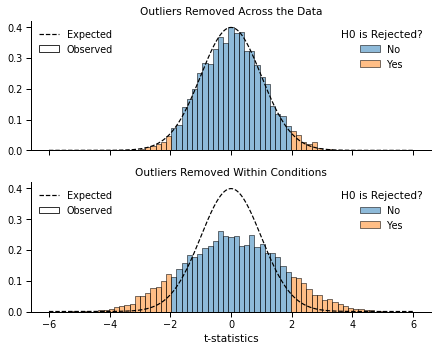## Figure 3: Understanding the magnifying impact of by-condition exclusions¶

Show Code
np.random.seed(2389326705)  # Seed for reproducibility

x, y = np.random.lognormal(size=(2, 100))  # Data before exclusions
xt = remove_outliers(x, cutoff_thresh=1.5)  # Data after exclusions
yt = remove_outliers(y, cutoff_thresh=1.5)

# Generating the "before exclusions" dataset, and flagging outliers in it.
resp = x.tolist() + y.tolist()
cond = np.repeat(["Control", "Treatment"], [x.shape, y.shape])
df_noexcl = pd.DataFrame(dict(Response=resp, Condition=cond))
df_noexcl["Outliers"] = df_noexcl.groupby("Condition").transform(
lambda x: flag_outliers_iqr(x, cutoff_thresh=1.5)
)

# Generating the "after exclusions" dataset
resp = xt.tolist() + yt.tolist()
cond = np.repeat(["Control", "Treatment"], [xt.shape, yt.shape])
df_excl = pd.DataFrame(dict(Response=resp, Condition=cond))
df_excl["Outliers"] = False
dfs = [df_noexcl, df_excl]

# Plotting the results
pal_rb = sns.color_palette()[0:5:3]
with sns.plotting_context("paper", font_scale=1.1):
pal = sns.color_palette()
fig, axes = plt.subplots(2, 2, figsize=(8, 6), sharey="row", sharex=True)
for ax, d in zip(axes, dfs):
sns.stripplot(
x="Condition",
y="Response",
hue="Outliers",
data=d,
alpha=0.75,
jitter=0.1,
order=["Control", "Treatment"],
ax=ax,
palette=pal_rb,
)
sns.despine()
ax.legend([], [], frameon=False)
ax.set_xlabel("")
ax.set_ylabel("")
for ax, d in zip(axes, dfs):
sns.pointplot(
x="Condition", y="Response", data=d, order=["Control", "Treatment"], ax=ax,
)
ax.set_ylabel("")
ax.set_xlabel("")

axes.set_title("Before Exclusions")
axes.set_title("After Exclusions")
axes.set_ylabel("Response")
axes.set_ylabel("Mean Response")

pval_before = stats.ttest_ind(x, y, equal_var=False).pvalue
pval_after = stats.ttest_ind(xt, yt, equal_var=False).pvalue
axes.annotate(f"p = {pval_before:.3f}", (0.5, 1.2), ha="center", va="center")
axes.annotate(f"p = {pval_after:.3f}", (0.5, 1.5), ha="center", va="center")
plt.savefig("Figures/Fig3.png", dpi=200, bbox_inches="tight")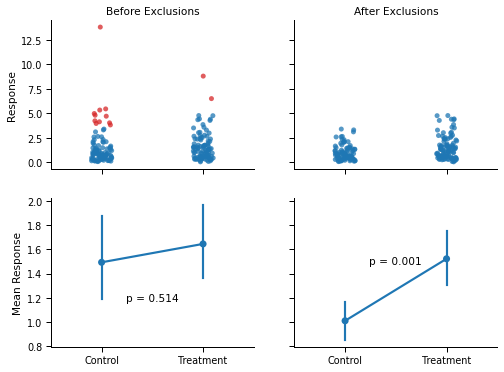## Figure 4: Simulations across different setups¶

Show Code
if "df_pvals" not in locals():  # See if the simulations are loaded in memory
try:  # If not, see if the simulations have been performed yet.
df_pvals = pd.read_csv("Buffer/Buffered_pvals.csv")
except:  # Otherwise perform them. Might take up to a few hours.
df_pvals = simulate_exclusion_impact(20000)
df_pvals.to_csv("Buffer/Buffered_pvals.csv", index=None)
with sns.plotting_context("paper", font_scale=1.2):
# Plot the simulation results
plot_simulation_results(df_pvals, include_iterated=False)
plt.tight_layout()
plt.savefig("Figures/Fig4.png", dpi=200, bbox_inches="tight")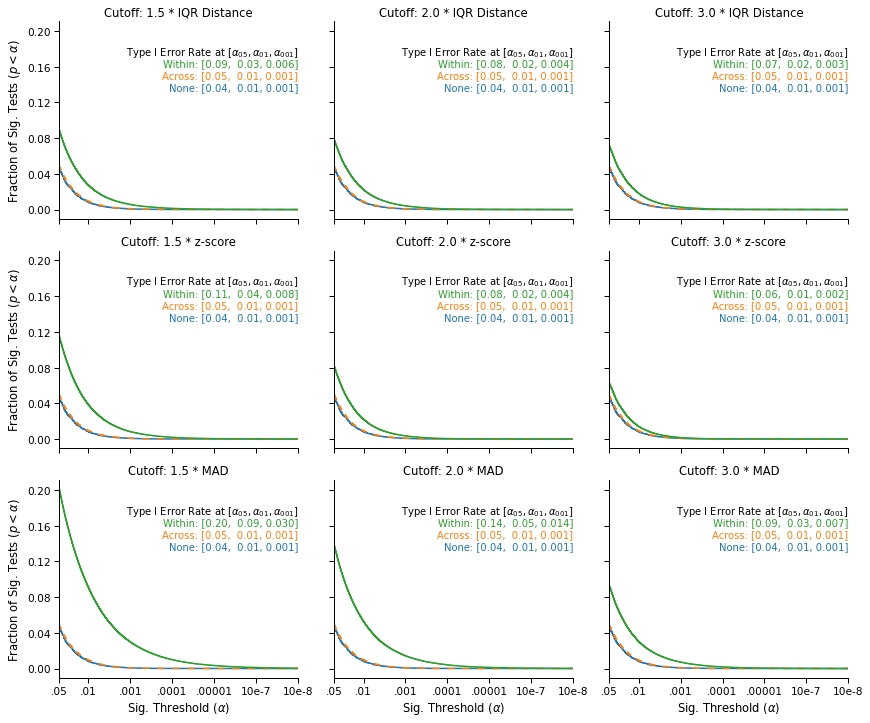### Subset Analysis: Type I Errors when applying a t-test to Log-Normal data¶

Show Code
with sns.plotting_context("paper", font_scale=1.2):
# Plot the simulation results
plot_simulation_results(
df_pvals.query("(Data == 'Log-Normal')").query('Test == "Welsch\'s t"'),
include_iterated=False,
)
plt.tight_layout()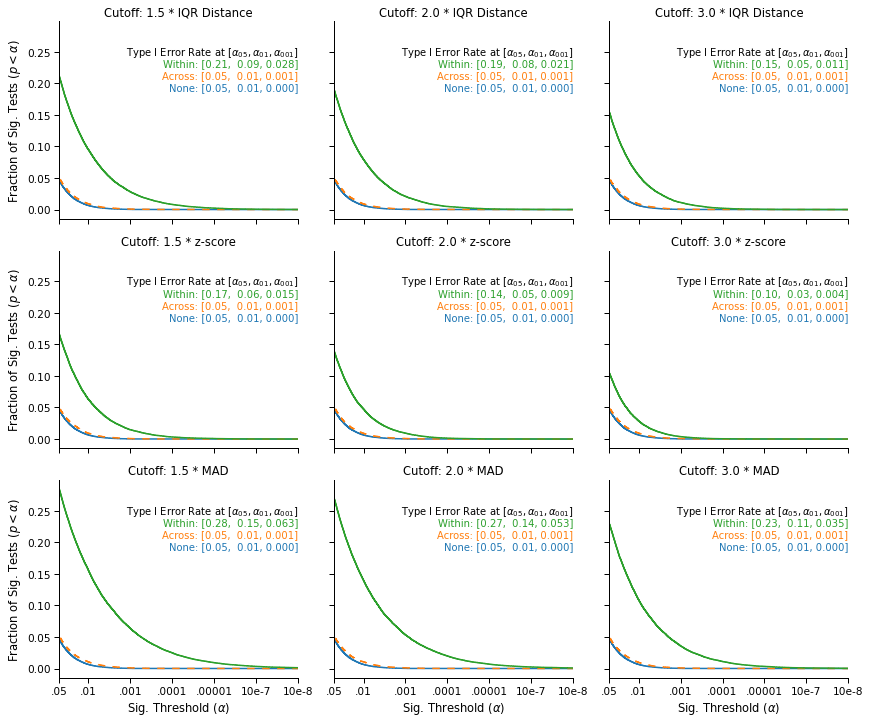### Granular description of Type I Error rates (α = .05) when excluding within conditions (nominal is 5%)¶

Show Code
gb = (
df_pvals.query("Level == 'Within'")
.groupby(["N", "Data", "Test", "Method", "Threshold"], sort=False)
.mean()
.iloc[:, 2]
.unstack(-2)
.unstack(-1)
* 100
)
gb.style.format("{:.0f}%")

Method IQR Distance z-score MAD
Threshold 1.5 2.0 3.0 1.5 2.0 3.0 1.5 2.0 3.0
N Data Test
50 Normal Welsch's t 7% 6% 5% 18% 11% 5% 23% 14% 6%
Mann-Whitney 7% 5% 5% 15% 9% 5% 20% 12% 6%
K-S 6% 5% 4% 12% 8% 4% 19% 11% 5%
Log-Normal Welsch's t 20% 18% 15% 15% 12% 10% 28% 26% 22%
Mann-Whitney 11% 10% 8% 9% 7% 6% 19% 17% 14%
K-S 10% 9% 7% 7% 6% 5% 17% 15% 12%
Normal Mixture Welsch's t 7% 6% 8% 10% 7% 8% 20% 11% 7%
Mann-Whitney 6% 5% 6% 9% 6% 6% 17% 10% 6%
K-S 6% 5% 5% 7% 5% 5% 16% 9% 5%
100 Normal Welsch's t 6% 5% 5% 17% 10% 5% 22% 12% 6%
Mann-Whitney 6% 5% 5% 15% 9% 5% 19% 11% 5%
K-S 5% 4% 3% 12% 8% 4% 19% 10% 5%
Log-Normal Welsch's t 21% 19% 15% 16% 13% 10% 28% 26% 23%
Mann-Whitney 11% 10% 8% 8% 7% 6% 19% 17% 13%
K-S 10% 9% 7% 7% 6% 5% 17% 15% 12%
Normal Mixture Welsch's t 7% 6% 8% 10% 7% 8% 20% 11% 6%
Mann-Whitney 6% 6% 6% 9% 6% 6% 17% 10% 6%
K-S 6% 5% 6% 7% 6% 5% 17% 9% 6%
250 Normal Welsch's t 6% 5% 5% 18% 11% 5% 23% 12% 5%
Mann-Whitney 6% 5% 5% 16% 9% 5% 20% 11% 5%
K-S 6% 5% 5% 13% 8% 5% 19% 11% 6%
Log-Normal Welsch's t 22% 19% 16% 19% 16% 11% 29% 28% 24%
Mann-Whitney 12% 10% 8% 9% 8% 6% 20% 18% 14%
K-S 10% 9% 8% 8% 7% 6% 18% 16% 12%
Normal Mixture Welsch's t 7% 6% 8% 9% 7% 9% 20% 11% 6%
Mann-Whitney 6% 5% 6% 8% 6% 6% 18% 9% 5%
K-S 6% 5% 6% 7% 5% 5% 17% 9% 5%

### Granular description of Type I Error rates (α = .01) when excluding within conditions (nominal is 10‰)¶

Show Code
gb = (
df_pvals.query("Level == 'Within'")
.groupby(["N", "Data", "Test", "Method", "Threshold"], sort=False)
.mean()
.iloc[:, 3]
.unstack(-2)
.unstack(-1)
* 1000
)
gb.style.format("{:.0f}‰")

Method IQR Distance z-score MAD
Threshold 1.5 2.0 3.0 1.5 2.0 3.0 1.5 2.0 3.0
N Data Test
50 Normal Welsch's t 18‰ 12‰ 10‰ 72‰ 34‰ 12‰ 117‰ 50‰ 14‰
Mann-Whitney 15‰ 10‰ 9‰ 57‰ 25‰ 10‰ 92‰ 37‰ 12‰
K-S 13‰ 8‰ 5‰ 36‰ 20‰ 7‰ 76‰ 34‰ 11‰
Log-Normal Welsch's t 85‰ 71‰ 46‰ 47‰ 37‰ 22‰ 147‰ 130‰ 100‰
Mann-Whitney 34‰ 28‰ 20‰ 21‰ 17‰ 12‰ 76‰ 67‰ 48‰
K-S 26‰ 23‰ 17‰ 16‰ 13‰ 10‰ 70‰ 57‰ 40‰
Normal Mixture Welsch's t 17‰ 14‰ 17‰ 31‰ 16‰ 18‰ 91‰ 39‰ 14‰
Mann-Whitney 14‰ 11‰ 11‰ 24‰ 13‰ 12‰ 69‰ 30‰ 12‰
K-S 13‰ 10‰ 11‰ 19‰ 11‰ 10‰ 61‰ 27‰ 11‰
100 Normal Welsch's t 16‰ 10‰ 9‰ 73‰ 34‰ 10‰ 111‰ 44‰ 12‰
Mann-Whitney 13‰ 10‰ 9‰ 58‰ 26‰ 10‰ 88‰ 34‰ 11‰
K-S 13‰ 10‰ 9‰ 36‰ 18‰ 10‰ 74‰ 29‰ 12‰
Log-Normal Welsch's t 95‰ 78‰ 55‰ 58‰ 43‰ 26‰ 152‰ 138‰ 107‰
Mann-Whitney 36‰ 29‰ 21‰ 21‰ 17‰ 12‰ 81‰ 68‰ 49‰
K-S 29‰ 24‰ 18‰ 17‰ 14‰ 11‰ 70‰ 58‰ 39‰
Normal Mixture Welsch's t 17‰ 15‰ 20‰ 31‰ 16‰ 21‰ 95‰ 39‰ 14‰
Mann-Whitney 14‰ 12‰ 12‰ 25‰ 14‰ 13‰ 76‰ 31‰ 13‰
K-S 12‰ 11‰ 13‰ 18‰ 12‰ 12‰ 61‰ 26‰ 12‰
250 Normal Welsch's t 16‰ 11‰ 10‰ 76‰ 36‰ 13‰ 112‰ 44‰ 13‰
Mann-Whitney 14‰ 10‰ 10‰ 60‰ 28‰ 11‰ 91‰ 35‰ 11‰
K-S 12‰ 9‰ 7‰ 40‰ 21‰ 10‰ 76‰ 32‰ 10‰
Log-Normal Welsch's t 103‰ 88‰ 61‰ 83‰ 62‰ 36‰ 164‰ 150‰ 117‰
Mann-Whitney 38‰ 31‰ 21‰ 25‰ 19‰ 13‰ 88‰ 75‰ 52‰
K-S 29‰ 24‰ 18‰ 18‰ 14‰ 10‰ 74‰ 59‰ 41‰
Normal Mixture Welsch's t 16‰ 14‰ 23‰ 28‰ 14‰ 24‰ 91‰ 34‰ 14‰
Mann-Whitney 13‰ 12‰ 13‰ 22‰ 13‰ 13‰ 73‰ 27‰ 12‰
K-S 12‰ 11‰ 12‰ 16‰ 10‰ 11‰ 58‰ 23‰ 11‰

### Granular description of Type I Error rates (α = .001) when excluding within conditions (nominal is 1‰)¶

Show Code
gb = (
df_pvals.query("Level == 'Within'")
.groupby(["N", "Data", "Test", "Method", "Threshold"], sort=False)
.mean()
.iloc[:, 4]
.unstack(-2)
.unstack(-1)
* 1000
)
gb.style.format("{:.1f}‰")

Method IQR Distance z-score MAD
Threshold 1.5 2.0 3.0 1.5 2.0 3.0 1.5 2.0 3.0
N Data Test
50 Normal Welsch's t 2.9‰ 1.2‰ 0.9‰ 19.9‰ 6.6‰ 0.9‰ 45.2‰ 12.1‰ 1.9‰
Mann-Whitney 1.6‰ 1.1‰ 0.8‰ 13.4‰ 4.4‰ 0.9‰ 29.2‰ 7.4‰ 1.4‰
K-S 2.1‰ 1.1‰ 0.7‰ 7.3‰ 2.6‰ 0.8‰ 20.2‰ 6.1‰ 1.4‰
Log-Normal Welsch's t 19.5‰ 14.4‰ 6.9‰ 7.6‰ 4.5‰ 2.3‰ 52.0‰ 43.0‰ 28.2‰
Mann-Whitney 5.8‰ 4.9‰ 3.7‰ 3.6‰ 2.4‰ 1.6‰ 18.9‰ 15.5‰ 9.8‰
K-S 4.8‰ 4.0‰ 3.1‰ 1.9‰ 1.7‰ 1.2‰ 17.3‰ 13.5‰ 7.8‰
Normal Mixture Welsch's t 2.8‰ 1.8‰ 1.9‰ 6.2‰ 2.1‰ 1.9‰ 33.5‰ 9.1‰ 2.1‰
Mann-Whitney 2.1‰ 1.4‰ 1.3‰ 4.2‰ 1.9‰ 1.4‰ 22.3‰ 6.2‰ 1.7‰
K-S 1.8‰ 1.6‰ 1.3‰ 2.9‰ 1.5‰ 1.3‰ 15.2‰ 4.5‰ 1.8‰
100 Normal Welsch's t 2.1‰ 1.1‰ 1.0‰ 20.8‰ 6.9‰ 1.1‰ 39.6‰ 11.3‰ 1.3‰
Mann-Whitney 1.6‰ 0.9‰ 0.8‰ 14.7‰ 4.2‰ 0.9‰ 28.6‰ 7.3‰ 1.1‰
K-S 1.3‰ 0.8‰ 0.7‰ 6.2‰ 2.4‰ 0.8‰ 17.6‰ 4.3‰ 1.0‰
Log-Normal Welsch's t 28.9‰ 21.8‰ 11.8‰ 13.1‰ 8.1‰ 3.4‰ 63.4‰ 53.6‰ 36.0‰
Mann-Whitney 7.0‰ 5.5‰ 3.0‰ 3.3‰ 2.4‰ 1.4‰ 24.8‰ 18.8‰ 11.9‰
K-S 4.8‰ 3.6‰ 2.6‰ 2.4‰ 1.8‰ 1.2‰ 19.8‰ 14.5‰ 8.7‰
Normal Mixture Welsch's t 2.3‰ 1.9‰ 2.7‰ 5.1‰ 2.1‰ 2.9‰ 32.7‰ 8.6‰ 2.1‰
Mann-Whitney 1.4‰ 1.2‰ 1.4‰ 3.8‰ 1.3‰ 1.6‰ 22.9‰ 6.0‰ 1.4‰
K-S 1.6‰ 1.1‰ 1.2‰ 2.4‰ 1.5‰ 1.2‰ 14.6‰ 3.9‰ 1.5‰
250 Normal Welsch's t 2.1‰ 1.4‰ 1.1‰ 21.8‰ 7.5‰ 1.6‰ 42.4‰ 9.8‰ 1.8‰
Mann-Whitney 1.4‰ 1.0‰ 1.0‰ 14.9‰ 4.6‰ 1.2‰ 30.0‰ 6.7‰ 1.2‰
K-S 1.4‰ 0.9‰ 0.9‰ 6.7‰ 2.4‰ 1.1‰ 18.6‰ 4.2‰ 1.2‰
Log-Normal Welsch's t 35.4‰ 26.3‰ 14.9‰ 23.4‰ 15.2‰ 5.7‰ 74.3‰ 62.8‰ 42.0‰
Mann-Whitney 7.6‰ 5.4‰ 2.8‰ 3.8‰ 2.6‰ 1.1‰ 28.3‰ 21.7‰ 12.8‰
K-S 4.7‰ 3.1‰ 1.9‰ 2.1‰ 1.2‰ 1.1‰ 20.3‰ 14.3‰ 8.2‰
Normal Mixture Welsch's t 1.9‰ 1.9‰ 3.6‰ 4.9‰ 1.9‰ 3.6‰ 30.8‰ 7.5‰ 1.9‰
Mann-Whitney 1.5‰ 1.4‰ 1.1‰ 3.4‰ 1.4‰ 1.2‰ 23.3‰ 5.3‰ 1.4‰
K-S 1.1‰ 0.9‰ 1.2‰ 2.2‰ 0.9‰ 0.9‰ 13.8‰ 4.0‰ 0.8‰

## Figure 5: Visualization of Results in Cao, Kong and Galinsky (2020)¶

Show Code
# Summary of means, by condition, study, and exclusion strategy.
with sns.plotting_context("paper", font_scale=1.1):
g = sns.catplot(
x="Condition",
y="ParetoEfficiency",
col="Exclusions",
col_wrap=2,
data=df_all,
kind="point",
hue="Study",
join=True,
dodge=True,
aspect=1.3,
height=4,
legend=False,
sharex=False,
hue_order=["Study 2", "Study 1"],
order=["No Eating", "Separate Eating", "Shared Eating"],
col_order=["Within, Iterated", "Within", "Across", "None"],
)

# Changing labels
g.set_titles("Exclusions: {col_name}")
g.set_xlabels("")

axes = g.axes.flatten()

# Adding p-values for study 1
for excl, ax in zip(["Within, Iterated", "Within", "Across", "None"], axes):
d = df1_subsets.query("Exclusions == @excl")
shared = d.query("Condition == 'Shared Eating'").ParetoEfficiency
sep = d.query("Condition == 'Separate Eating'").ParetoEfficiency
no = d.query("Condition == 'No Eating'").ParetoEfficiency
p1 = stats.ttest_ind(sep, no, equal_var=False).pvalue
p2 = stats.ttest_ind(shared, sep, equal_var=False).pvalue
ax.annotate(
f"Separate vs. No\np = {p1:-1.3f}",
(0.5, 850),
ha="center",
va="center",
color=pal,
)
ax.annotate(
f"Shared vs. Separate\np = {p2:.3f}",
(1.6, 850),
ha="center",
va="center",
color=pal,
)

# Adding p-values for study 2
for excl, lab, ax in zip(
["Within, Iterated", "Within", "Across", "None"],
[
"Excluded Within Conditions, Iteratively",
"Excluded Within Conditions, Once",
"Excluded Across the Data",
"No Exclusions",
],
axes,
):
d = df2_subsets.query("Exclusions == @excl")
shared = d.query("Condition == 'Shared Eating'").ParetoEfficiency
sep = d.query("Condition == 'Separate Eating'").ParetoEfficiency
no = d.query("Condition == 'No Eating'").ParetoEfficiency
p1 = stats.ttest_ind(sep, no, equal_var=False).pvalue
p2 = stats.ttest_ind(shared, sep, equal_var=False).pvalue
ax.set_ylim(780, 1030)
ax.set_title(lab, size=12)
ax.annotate(
f"Separate vs. No\np = {p1:-1.3f}",
(0.5, 1010),
ha="center",
va="center",
color=pal,
)
ax.annotate(
f"Shared vs. Separate\np = {p2:.3f}",
(1.5, 1010),
ha="center",
va="center",
color=pal,
)
g.add_legend(title="", loc=(0.1, 0.7))
plt.savefig("Figures/Fig5.png", dpi=200, bbox_inches="tight")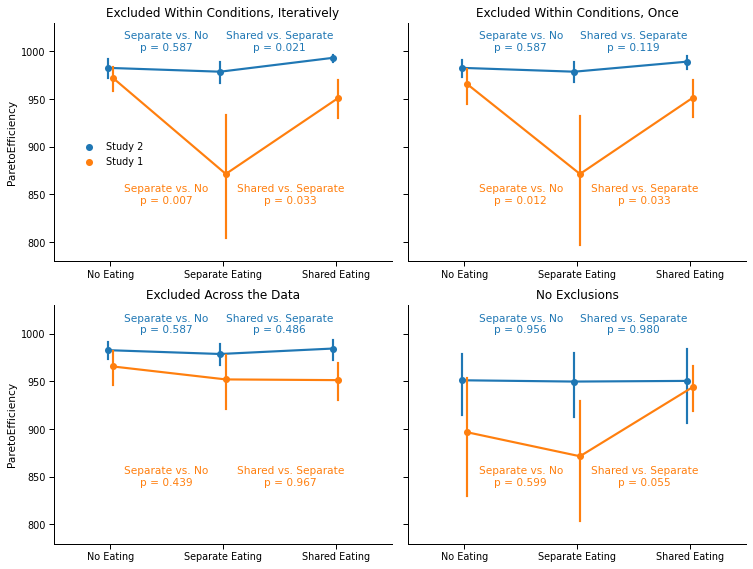## Figure 6: Simulating Exclusion Impacts in Cao, Kong and Galinsky (2020)¶

### In Study 2¶

Show Code
if (
"df_pvals_ckg_s2" not in locals()
):  # See if the simulations for CKG are loaded in memory
try:  # If not, see if the simulations have been performed yet.
df_pvals_ckg_s2 = pd.read_csv("Buffer/Buffered_pvals_ckg_s2.csv")
except:  # Otherwise perform them.
df_pvals_ckg_s2 = simulate_exclusion_impact_ckg(
df2_clean.sort_values("Condition"), 20000, n=31
)
df_pvals_ckg_s2.to_csv("Buffer/Buffered_pvals_ckg_s2.csv", index=None)

# Plot the simulation results
with sns.plotting_context("paper", font_scale=1.2):
plot_simulation_results(df_pvals_ckg_s2, include_iterated=True)
plt.tight_layout()
plt.savefig("Figures/Fig6.png", dpi=200, bbox_inches="tight")